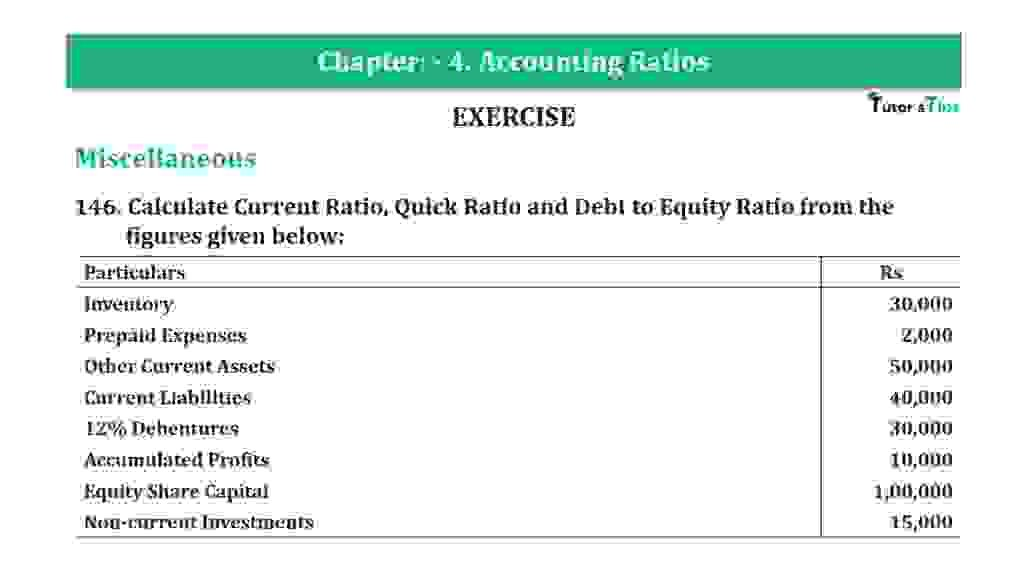# Question 146 Chapter 4 of +2-B – T.S. Grewal 12 ClassQuestion 146 Chapter 4 of +2-B

Miscellaneous

146. Calculate Current Ratio, Quick Ratio and Debt to Equity Ratio from the
figures given below:

 Particulars Rs Inventory 30,000 Prepaid Expenses 2,000 Other Current Assets 50,000 Current Liabilities 40,000 12% Debentures 30,000 Accumulated Profits 10,000 Equity Share Capital 1,00,000 Non-current Investments 15,000

### The solution of Question 146 Chapter 4 of +2-B: –

I

 Current Assets = Inventory + Prepaid Expenses + Other Current Assets = Rs 30,000 + Rs 2,000 + Rs 50,000 = Rs 82,000 Current Liabilities = Rs 40,000
 Current Ratio = Current Assets Current Liabilities
 Current Ratio = Rs. 82,000 Rs 40,000 = 2.05 : 1

II

 Liquid Assets = Current Assets − Inventory − Prepaid Expenses = Rs 82,000 − Rs 30,000 − Rs 2,000 = Rs 50,000
 Liquid Ratio = Liquid Assets Current Liabilities
 Liquid Ratio = Rs. 50,000 Rs 40,000 = 1.25 : 1

III

 Long term Debts = Rs 30,000 Equity = Accumulated Profits + Equity Share Capital = Rs 10,000 + Rs 1,00,000 = Rs 1,10,000
 Debt Equity Ratio = Debts Equity
 Debt Equity Ratio = Rs. 30,000 Rs 1,10,000 = 0.27 : 1

Thanks, Please Like and share with your friends

Comment if you have any question.

Also, Check out the solved question of previous Chapters: –

### T.S. Grewal’s Double Entry Book Keeping (Vol. II: Accounting for Companies)T.S. Grewal’s Analysis of Financial Statements